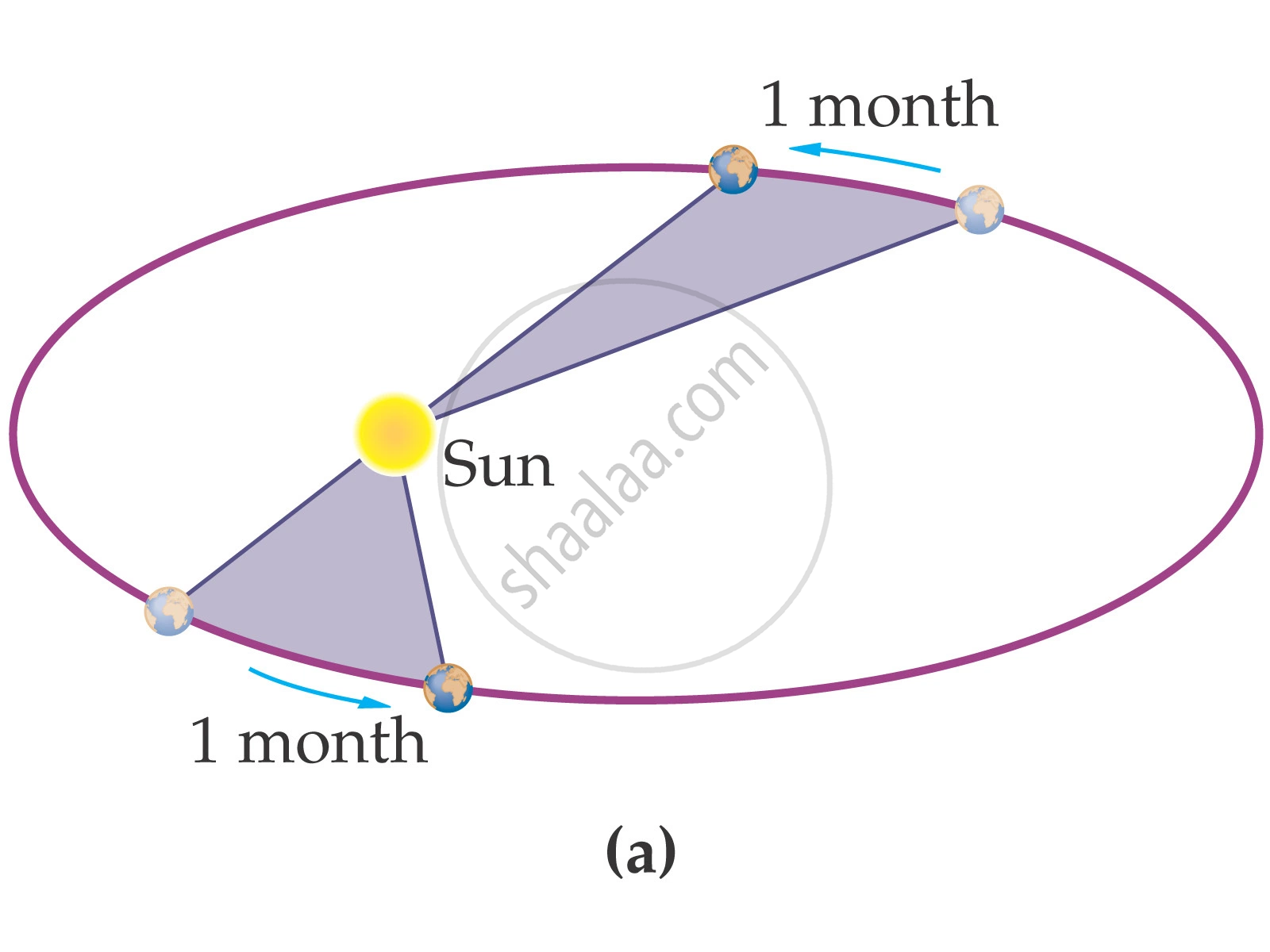# Torque and Angular Momentum

#### description

• Moment of a Force (Motion of System of Particles and Rigid Body)
• Angular Momentum and Law of Conservation of Angular Momentum and Its Applications
• Moment of force (Torque)
• Angular momentum of a particle
• Torque and angular momentum for a system of particles
• conservation of angular momentum

### Torque & Angular Momentum:

• The rotational analogue of force is moment of force (Torque).

• If a force acts on a single particle at a point P whose position with respect to the origin 'O' is given by the position vector r the moment of the force acting on the particle with respect to the origin 'O' is defined as the vector product t = r × F = rF sinΘ

• Torque is vector quantity.

• The moment of a force vanishes if either

• The magnitude of the force is zero, or

• The line of action of the force (r sinΘ) passes through the axis.

Example: Determine the torque on a bolt, if you are pulling with a force F directed perpendicular to a wrench of length 'l'cm?

Solution: t = r x F = rF sinΘ

In this case Θ=90o

The quantity angular momentum is the rotational analogue of linear momentum.

• It could also be referred to as moment of (linear) momentum.

• l = r × p

• Rotational analogue of Newton’s second law for the translational motion of a single particle: "dl"/"st" = tau

Torque and angular momentum of system of particles:

• The total angular momentum of a system of particles about a given point is addition of the angular momenta of individual particles added vectorially.

"L" ="l"_1 + "l"_2 + ... + "l"_"n" = sum_(1)^nl_i             where  l_i=r_ixp_i

Similarly for total torque on a system of particles is addition of the torque on an individual particle added vectorially.

tau=sum_(1)^nr_i xx F_i = tau_"ext" + tau_"int"

• The torque resulting from internal forces is zero, due to

• Newton’s third law i.e. these forces are equal and opposite.

• These forces act on the line joining any two particles

• The time rate of the total angular momentum of a system of particles about a point is equal to the sum of the external torques acting on the system taken about the same point.

"dL"/"dt" = tau_"ext"

Conservation of Angular Momentum

If the external torque acting on a system is zero, then its angular momentum remains conserved.
If text = 0, then dL/dt = 0 = I(ω) = constant ⇒ I1ω1= I2ω2If you would like to contribute notes or other learning material, please submit them using the button below.

#### Video Tutorials

We have provided more than 1 series of video tutorials for some topics to help you get a better understanding of the topic.

Series 1

Series 2

### Shaalaa.com

torque and radius of gyration [00:16:57]
S
0%